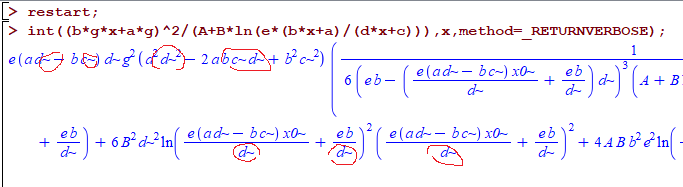# Question:int displays large output on the screen on same command first and second time only in Maple 2023?

## Question:int displays large output on the screen on same command first and second time only in Maple 2023?

Maple 2023

Do others see this problem? I do not understand what is going on. I am seeing this problem on many integrals

```restart;
int(integrand)
#Large output displayed
#echo the input
int(...)
#Large output displayed
#echo the input
int(...)
#echo the input only. Large output gone
int(...)
#echo the input only. Large output gone

restart;
int(...)
#Large output displayed
#echo the input
int(...)
#echo the input only. Large output gone
int(...)
#echo the input only. Large output gone

```

In all the above, it is the same command used.

i.e. first time (sometimes needs two times), Maple displays large out. But looking at the end of this output, the very last line, we see the same integral/command is returned.

But second time and any attempt after that, it no longer gives that large output, but returns back/echos the command on the screen only.

Attached is worksheet showing this. This is new behaviour in Maple 2023 and I am baffled by it. Do others see it? Why does it happen. I will report it if others confirm it. I just wanted to make sure first it is not just me seeing this.

Is it possible the large output is side effect and is being printed by error to the screen by internal Maple code? But why does it stop the second/third time?

 > interface(version);> restart;
 > int((b*g*x+a*g)^2/(A+B*ln(e*(b*x+a)/(d*x+c))),x)> int((b*g*x+a*g)^2/(A+B*ln(e*(d*x+c)/(b*x+a))),x)

Warning, if e is meant to be the exponential e, use command/symbol completion or palettes to enter this special symbol, or use the exp functionWarning, if e is meant to be the exponential e, use command/symbol completion or palettes to enter this special symbol, or use the exp functionWarning, if e is meant to be the exponential e, use command/symbol completion or palettes to enter this special symbol, or use the exp functionWarning, if e is meant to be the exponential e, use command/symbol completion or palettes to enter this special symbol, or use the exp functionWarning, if e is meant to be the exponential e, use command/symbol completion or palettes to enter this special symbol, or use the exp function> int((b*g*x+a*g)^2/(A+B*ln(e*(d*x+c)/(b*x+a))),x)> int((b*g*x+a*g)^2/(A+B*ln(e*(d*x+c)/(b*x+a))),x)> int((b*g*x+a*g)^2/(A+B*ln(e*(d*x+c)/(b*x+a))),x)> restart;
 > int((b*g*x+a*g)^2/(A+B*ln(e*(d*x+c)/(b*x+a))),x)

Warning, if e is meant to be the exponential e, use command/symbol completion or palettes to enter this special symbol, or use the exp functionWarning, if e is meant to be the exponential e, use command/symbol completion or palettes to enter this special symbol, or use the exp functionWarning, if e is meant to be the exponential e, use command/symbol completion or palettes to enter this special symbol, or use the exp functionWarning, if e is meant to be the exponential e, use command/symbol completion or palettes to enter this special symbol, or use the exp functionWarning, if e is meant to be the exponential e, use command/symbol completion or palettes to enter this special symbol, or use the exp function> int((b*g*x+a*g)^2/(A+B*ln(e*(d*x+c)/(b*x+a))),x)>
 >

Update

Do you want to see something more bizzar? Try this command on same integral

```restart;
res:=int((b*g*x+a*g)^2/(A+B*ln(e*(b*x+a)/(d*x+c))),x,method=_RETURNVERBOSE)
```

It prints to the screen results with ~ all over. But this seems to be internal leaked output and not part of the actual output returned.By issuing the command as follows instead

```restart;
res:=int((b*g*x+a*g)^2/(A+B*ln(e*(b*x+a)/(d*x+c))),x,method=_RETURNVERBOSE):
```

notice the at the end!  I still see the same output as above printed displayed.

This tells me this is a leaked printout from an internal integration function.

Could others confirm this?﻿